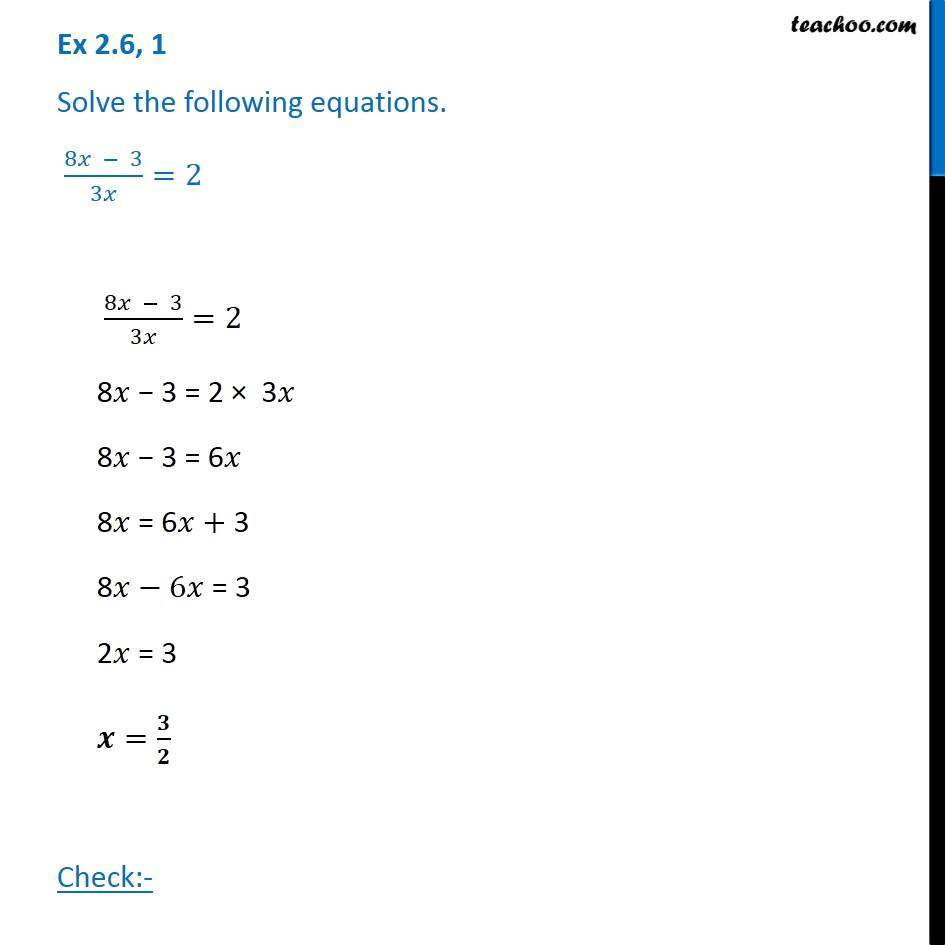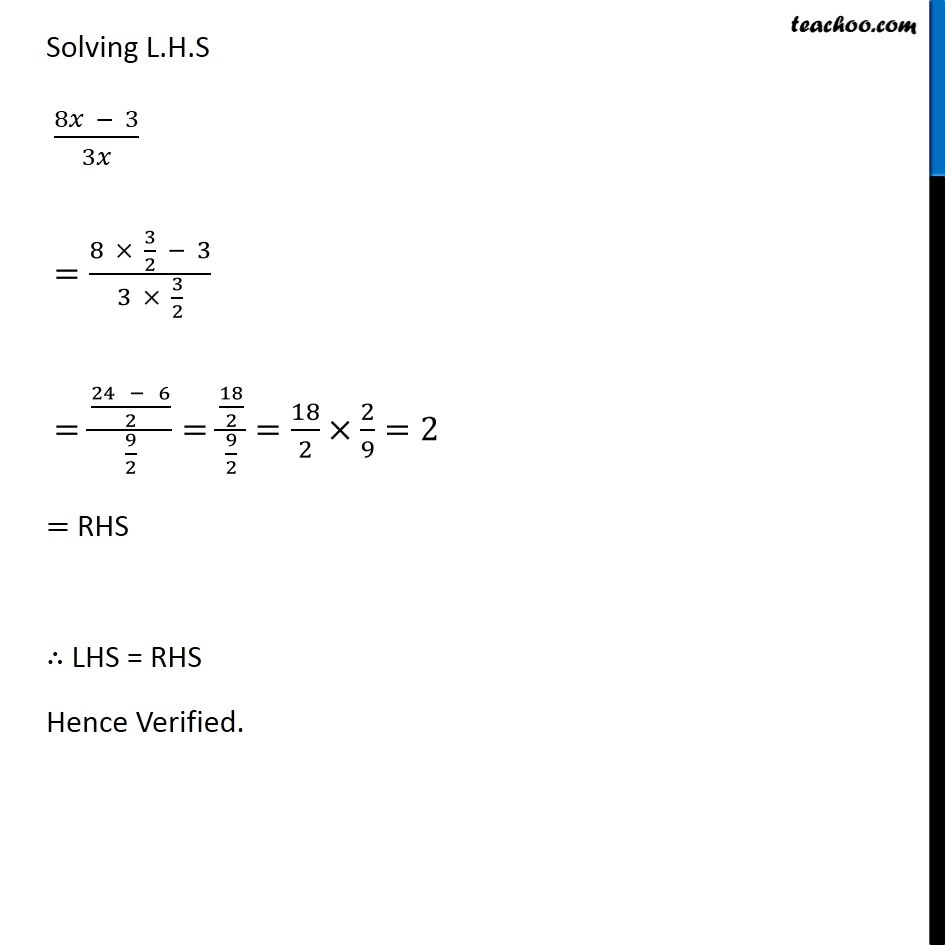Solving difficult equations - Word Problems

Chapter 2 Class 8 Linear Equations in One Variable
Serial order wiseLearn in your speed, with individual attention - Teachoo Maths 1-on-1 Class

### Transcript

Question 1 Solve the following equations. (8𝑥 − 3)/3𝑥=2 (8𝑥 − 3)/3𝑥=2 8𝑥 − 3 = 2 × 3𝑥 8𝑥 − 3 = 6𝑥 8𝑥 = 6𝑥+ 3 8𝑥−6𝑥 = 3 2𝑥 = 3 𝒙=𝟑/𝟐 Check:- Solving L.H.S (8𝑥 − 3)/3𝑥 =(8 × 3/2 − 3)/(3 × 3/2) =((24 − 6)/2)/(9/2)=(18/2)/(9/2)=18/2×2/9=2 = RHS ∴ LHS = RHS Hence Verified.